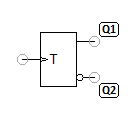# T flip-flopThe T flip-flop (toggle flip-flop) has only one input T. If there is a positive edge at input T from 0 to 1, the outputs change (toggle) their states. The initial state of the outputs when the supply voltage is applied is random. However, Q1 = not Q2 always applies.

Truth table

T Q1 Q2
q2 q1
x q1 q2

x = any (0 or 1) ↑ = positive edge from 0 to 1 q = state of output Q before the edge